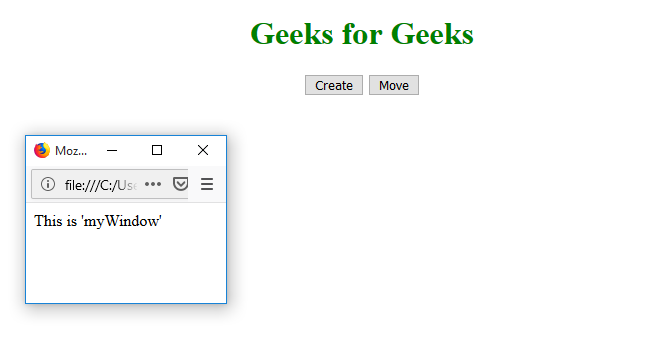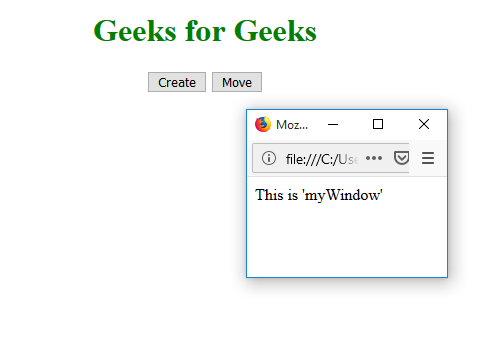# HTML | Window moveTo() Method

• Difficulty Level : Basic
• Last Updated : 04 Aug, 2022

The moveTo() method is used in the window to moves the window from the left and top coordinates.

Syntax:

`window.moveTo(x, y)`

Parameter:

• x: A positive or negative number that specifies the horizontal coordinate to be moved to
• y: A positive or negative number specifies the vertical coordinate to be moved to

Example: Move the window.

## html

 ```<``html``>``<``head``>``    ``<``title``>``      ``Window moveTo() Method``    ````    ``<``style``>``        ``h1 {``            ``color: green;``        ``}``    ````` `<``body``>``    ``<``center``>``        ``<``h1``>Geeks for Geeks``        ``<``button` `onclick``=``"openWin()"``>``          ``Create``      ````        ``<``button` `onclick``=``"moveWin()"``>``          ``Move``      ``` `        ``<``script``>``            ``var myWindow;` `            ``function openWin() {``                ``myWindow =``                  ``window.open("",``                              ``"myWindow",``                              ``"width=200",``                              ``"height=100");``              ` `                ``myWindow.document.write(``                  ``"``<``p``>This is 'myWindow'``");``            ``}``            ``function moveWin() {``                ``myWindow.moveTo(500, 100);``                ``myWindow.focus();``            ``}``        ````    ```````

Output:

Initial:New window:Move window:Supported Browsers: The browser supported by Window moveTo() Method are listed below: